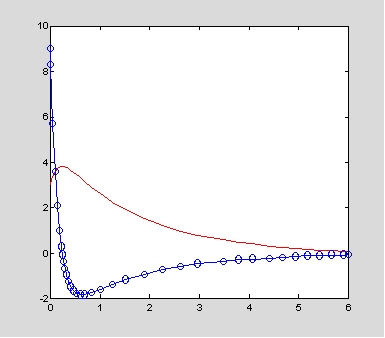## 12/22/2006

### 12.8非線性方程式解

`　V=πR²H/3`

`Q = Cd A (2gh)½`

dV/dt = Qin-Q

dV/dt=d/dt[πr²dh/3]=πR²h²/(3H²]dh/dt ，　故

[πR²h²/(3H²]dh/dt=Qin-Cd*A*(2gh)½　　整理之，

dh/dt=[Qin-Cd*A*(2gh)½]/[πR²h²/(3H²]

`function [h,t]=tri_tank(tf,h0,R,H,cd,A,Qin)% Using Euler method to solve differential eqs.%  Water tank% Inputs:%    h0:initial water level%    tf:time interval[t1 t2]%    R,H:radius & height of tank, m%    cd:coefficient of orifice%    A: Crosssection of orifice, m^2%    Qin: Inlet flow, cms% Example: h=tri_tank([0 10],9,10,9,0.6,1,10)aa=3*(H/R)^2/pi;g=9.8;vv=@(t,h) aa*(Qin-cd*A*sqrt(2*g*h));[t,h]=ode23(vv,tf,h0);plot(t,h,'r-',t,h,'.');xlabel('t'),ylabel('y');`

`>>hold on; for i=[5 7 10 15],h=tri_tank([0 10],9,10,9,0.6,1,i);end`### 高階微分方程式

`　　5y"+7y'+4y = f(t)`

`　　y"=(1/5)f(t)-(4/5)y-(7/5)y'`

`  x1=y  x2=y'`

`  x1'=y'  x2'=y"`

`  x1'=x2  x2'=y"=(1/5)f(t)-(4/5)x1-(7/5)x2`

`fun=@(t,x) [x(2);(1/5)*sin(t)-4*x(1)-7*x(2)];[t,x]=ode23(fun,[0 6],[3 9]);plot(t,x(:,1),'r-',t,x(:,2),'bo',t,x(:,2))`